# Revisit Poisson spiking LIF model #1487

Open
opened this issue Nov 7, 2018 · 8 comments
Open

# Revisit Poisson spiking LIF model#1487

opened this issue Nov 7, 2018 · 8 comments
Labels

###arvoelke commented Nov 7, 2018 • edited

 Related: I think we should revisit this old issue / PR of including Poisson spiking models within Nengo. There is a nice mathematical argument for why the Poisson spiking version of the LIF model is superior to its regular spiking LIF counterpart. I have some math in my upcoming thesis that shows our LIF model is actually systematically biased to incorrectly represent high-frequency signals, even after washing out the initial transient (#1415). And especially as the spike rates are lowered relative to the frequency of the signal. There will always be some global error due to a systematic shift from some ideal state that I've quantified. Intuitively, this arises from the neurons not being "uniformly ready to spike" at any given moment in time. No amount of scaling `n_neurons` can correct for this bias (see plot at bottom of post for experimental validation, noting that the performance plateaus for the `LIF` model)! However, Poisson spiking can! The following `PoissonLIF()` is actually optimal in the sense of expectation, as the expected filtered error theoretically scales to `0` as `n_neurons -> infinity`, regardless of the input signal frequency. The memoryless feature of the exponential distribution (the time between Poisson events) is what gives us this property. Even more strongly, this guarantees that there is no approximation being made when switching between filtered `LIFRate()` and filtered `PoissonLIF()`, apart from variability in the PSC (which again scales to `0`). But this comes at the cost of increased variability for lower numbers of neurons and constant inputs, compared to `LIF()`. I have a currently-unpublished proof of this in my upcoming thesis and in discussions with @celiasmith. Some drawbacks: Time-to-first-spike is now probabilistic (e.g., consider rank-order encoding, or WTA mutual inhibition). No notion of resetting the voltage to zero via inhibition. Can violate its own refractory period (with small probability). Higher variability for constant inputs. Some advantages: Better scaling guarantees (see two figures below). Potentially faster. Less memory. No initial transient at the start of the simulation (see #1415). No latency for discontinuous jumps in input (related to previous point, and higher frequencies in general). Here is some experimental validation, with elements of the code borrowed from @tbekolay and @tcstewar: ```class PoissonLIF(LIFRate): """Poisson-spiking leaky integrate-and-fire (LIF) neuron model. Parameters ---------- tau_rc : float Membrane RC time constant, in seconds. Affects how quickly the membrane voltage decays to zero in the absence of input (larger = slower decay). tau_ref : float Absolute refractory period, in seconds. This is how long the membrane voltage is held at zero after a spike. amplitude : float Scaling factor on the neuron output. Corresponds to the relative amplitude of the output spikes of the neuron. """ probeable = ('spikes',) def __init__(self, tau_rc=0.02, tau_ref=0.002, amplitude=1, seed=None): super(PoissonLIF, self).__init__( tau_rc=tau_rc, tau_ref=tau_ref, amplitude=amplitude) # TODO(arvoelke): the simulator should pass in the rng self.rng = np.random.RandomState(seed=seed) def _sample_exponential(self, rates): # generate an exponential random variable (time between # poisson events) using its inverse CDF. note that this # distribution is "memoryless", which is why we don't # need to save this state or worry about what happens # outside of this time-step. return -np.log1p(-self.rng.rand(len(rates))) / rates def _poisson_step_math(self, dt, rates, spiked): spiked[...] = 0 next_spikes = np.zeros_like(spiked) to_process = np.ones_like(spiked, dtype=bool) while np.any(to_process): next_spikes[to_process] += self._sample_exponential( rates[to_process]) to_process &= next_spikes < dt spiked[to_process] += self.amplitude / dt def step_math(self, dt, J, spiked): rates = np.zeros_like(J) LIFRate.step_math(self, dt=1, J=J, output=rates) self._poisson_step_math(dt, rates, spiked)``` ```from collections import defaultdict import nengo import numpy as np import matplotlib.pyplot as plt import seaborn as sns from pandas import DataFrame def go(freq, neuron_type, n_neurons_over_freq=50, # scale-invariant n_steps=1000, tau_times_freq=0.01, # dimensionless dt_times_freq=0.001, # dimensionless max_rates=nengo.dists.Uniform(20, 40), seed=0, ): n_neurons = int(n_neurons_over_freq * freq) tau = tau_times_freq / freq dt = dt_times_freq / freq with nengo.Network(seed=seed) as model: u = nengo.Node(lambda t: np.sin(freq*2*np.pi*t)) x = nengo.Ensemble(n_neurons, 1, neuron_type=neuron_type, max_rates=max_rates) nengo.Connection(u, x, synapse=None) p_actual = nengo.Probe(x, synapse=tau) p_ideal = nengo.Probe(u, synapse=tau) with nengo.Simulator(model, dt=dt) as sim: if isinstance(neuron_type, nengo.LIF): # https://github.com/nengo/nengo/issues/1415 # uniform is a decent approximation for the voltage # after the mixing time sim.signals[sim.model.sig[x.neurons]['voltage']] = ( np.random.RandomState(seed=seed).rand(n_neurons)) # For some extra assurances, run # a bit to give each neuron time to spike or settle sim.run(1. / np.max(sim.data[x].max_rates)) # Then clear that probe data # https://github.com/nengo/nengo/issues/963 for probe in sim.model.probes: sim._probe_outputs[probe] = [] sim.run_steps(n_steps) return nengo.utils.numpy.rmse( sim.data[p_actual], sim.data[p_ideal])``` ```data = defaultdict(list) for neuron_type in (nengo.neurons.PoissonLIF(), nengo.neurons.LIF()): for freq in np.linspace(1, 101, 11): for seed in range(5): data['Model'].append(type(neuron_type).__name__) data['Frequency'].append(freq) data['Seed'].append(seed) data['RMSE'].append(go(freq, neuron_type, seed=seed)) plt.figure(figsize=(14, 6)) sns.lineplot(data=DataFrame(data), x="Frequency", y="RMSE", hue="Model") plt.show() ```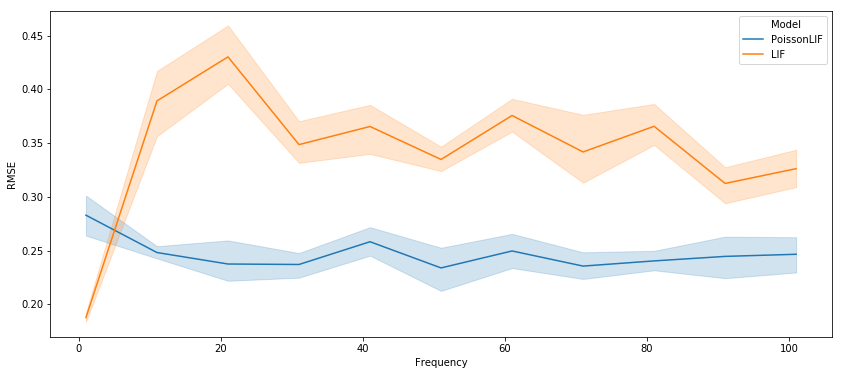```data = defaultdict(list) for neuron_type in (nengo.neurons.PoissonLIF(), nengo.neurons.LIF()): for n_neurons_over_freq in np.linspace(10, 501, 11): for seed in range(5): freq = 10 data['Model'].append(type(neuron_type).__name__) data['# Neurons'].append(n_neurons_over_freq * freq) data['Seed'].append(seed) data['RMSE'].append(go(freq, neuron_type, n_neurons_over_freq=n_neurons_over_freq, seed=seed)) plt.figure(figsize=(14, 6)) sns.lineplot(data=DataFrame(data), x="# Neurons", y="RMSE", hue="Model") plt.xscale('log') plt.show()```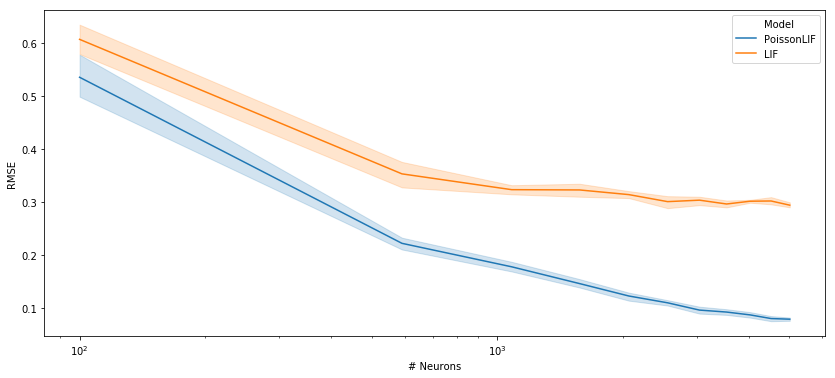###hunse commented Nov 8, 2018

 I'm not necessarily saying this shouldn't be in Nengo, but my go-to for new things these days is `nengo_extras`. You can put it there, and then people can try it out, while not making it quite as big of a commitment. That said, I think this would be the first neuron model we have with stochasticity in it, so we may need some changes to this repository to support it (e.g. the `step` function will need a RNG, and ideally IMO this would be the simulator RNG so that it's easy to make simulations reproducible and to change the randomness without rebuilding the model).

###arvoelke commented Nov 12, 2018 • edited

 In addition to the `rng` considerations, another reason it might be useful to consider this for Nengo core is it could become an Ensemble-level config flag that applies to all neurons, whether they be `Sigmoid`, `RectifiedLinear`, or whatever. The method only needs a static non-negative response curve (i.e., `rates` method with respect to `J`) in order to derive the `step_math` function. Similar to what @tbekolay implemented in #1225. This might also promote better interoperability with `nengo_dl`, for the use case where one might like to train with `rates` and test with Poisson spiking.

###arvoelke commented Nov 14, 2018 • edited

 Quick update here. I tried to battle-test this a bit by running it against the suite of nengo-benchmarks. See #1482 (comment) for a testing script that requires minimal changes. But the only ones that report `rmse` are: ``````benchmarks = [ nengo_benchmarks.CommunicationChannel, nengo_benchmarks.CircularConvolution, nengo_benchmarks.MatrixMultiply, ] `````` and all of these supply constant inputs to the model, which means we are operating at the zero-frequency point (the left-most point in the first plot above), where regular spiking has less variability due to its periodic output. The firing rates are also already very high with the defaults. And so it's not surprising that `PoissonLIF` does worse on these benchmarks. The only benchmark where I might expect an improvement is the `Oscillator`, but that does not report accuracy. To see the full potential, I'd want to use `nengolib`'s discrete Principle 3 mapping, at low firing rates, and compare the output of the recurrent synapse to the ideal representation.

###arvoelke commented Nov 14, 2018 • edited

 All considered, I think my vote goes for not including this in Nengo at the moment, and instead making a separate issue/PR for adding `rng` as an optional argument to `step_math`, which requires minimal work, as this was a part of #1225. I can Poisson-ize a bunch of the models and put them in nengolib until this is proved out a bit. And I can forsee other benefits to giving neuron models direct access to random numbers, e.g., experimenting with new models, or emulating special hardware features.

###HugoChateauLaurent commented Feb 8, 2019

 Your implementation does not seem to spike at the correct firing rate when I test it (see below).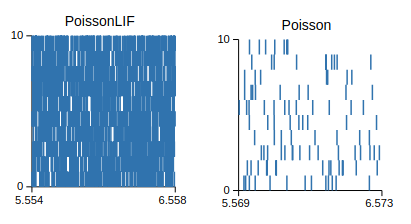``````nbSim = 10 PoissonLIF = nengo.Ensemble(int(nbSim), 1, encoders=np.ones((int(nbSim),1)), gain=np.ones((int(nbSim))), bias=np.zeros((int(nbSim))), neuron_type=PoissonLIF()) Poisson = nengo.Ensemble(int(nbSim), 1, encoders=np.ones((int(nbSim),1)), gain=np.ones((int(nbSim))), bias=np.zeros((int(nbSim))), neuron_type=nengo.neurons. rate = nengo.Node([10,10]) # we want it to fire at 10Hz nengo.Connection(rate,PoissonLIF) nengo.Connection(rate,Poisson) `````` I added a simpler Poisson generator with more extensive firing rate testings here: #1505.

###arvoelke commented Feb 8, 2019 • edited

 The difference between our implementations has to do with how tuning curves are defined in Nengo, and to be consistent with `nengo.LIF()`. When you give a gain of 1 and a bias of 0, an input current of 10 does not induce a firing rate of 10 Hz in the `LIF` model (although it does if you are using the `nengo.SpikingRectifiedLinear()` model). This is because, at the level of gains and biases, Nengo is working in current space, not activity space. The goal here was to get tuning curves that are identical to `LIF` (in the sense of expected values). Here is a test that shows this is working as intended. I set the `max_rates` of the tuning curves to `desired_rate` and then simulate them at that part of the curve. Replacing `PoissonLIF()` with `nengo.LIF()` gives exactly `desired_rate`, and so the two models are consistent with one another. ```desired_rate = 42 sim_t = 10.0 n_neurons = 256 neuron_type = PoissonLIF() # nengo.LIF() with nengo.Network() as model: u = nengo.Node(1) x = nengo.Ensemble(n_neurons, 1, encoders=np.ones((n_neurons, 1)), max_rates=[desired_rate]*n_neurons, neuron_type=neuron_type) nengo.Connection(u, x, synapse=None) p = nengo.Probe(x.neurons, 'spikes', synapse=None) with nengo.Simulator(model) as sim: sim.run(sim_t) print(np.count_nonzero(sim.data[p]) / sim_t / n_neurons)``` `>>> 41.245...`

###arvoelke commented Feb 13, 2019 • edited

 It also occurred to me that Nengo's `SpikingRectifiedLinear` model already shares the properties that we desire from Poisson-spiking models, namely scaling correctly with input frequency and neuron count. Assuming the initial voltages are uniformly distributed (the neuronal states are already "mixed"), via: ``` with nengo.Simulator(model) as sim: # https://github.com/nengo/nengo/issues/1415 sim.signals[sim.model.sig[x.neurons]['voltage']] = ( rng.rand(x.n_neurons)) ...``` then, due to the way the input current is clipped at 0, the population's state will trivially remain in a uniform distribution, no matter what you do to it. My 'secret' math then says this satisfies sufficient criteria for zero-mean error (taking the expectation across the pool of neurons), as all of the neurons will always be "ready to spike" with the correct (uniform) probability.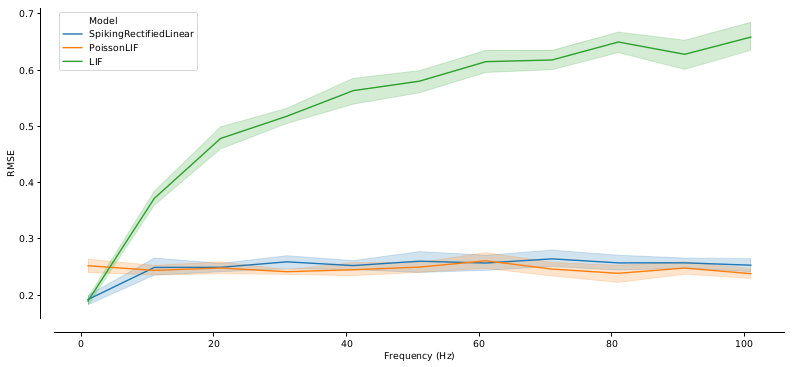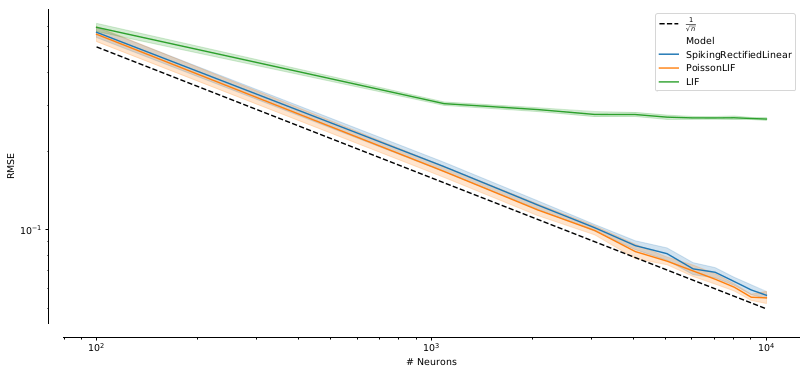Basically, LIF approaches maximum error rates with higher frequencies, and plateaus in performance with higher neuron counts (for any non-zero input frequency). On the other hand, neither Poisson-spiking nor (non-leaky) integrate-and-fire models have this issue (they are both frequency-invariant, and continue to scale as `O(1/sqrt(n))` for increasingly-large `n`). Details of these results are to be published very soon.
###arvoelke commented Jul 19, 2019 • edited
 Here is a simpler implementation that should also be much faster in the event that many spikes happen per time-step (substitute this `step_math` method for the one in #1487 (comment)): ``` def step_math(self, dt, J, spiked): rates = np.zeros_like(J) LIFRate.step_math(self, dt=1, J=J, output=rates) n_spikes = self.rng.poisson(rates * dt, spiked.size) spiked[...] = self.amplitude * n_spikes / dt``` Rather than count the number of exponential events one at a time, this uses a set of approximations that iterate much faster for large `n_spikes`: https://github.com/numpy/numpy/blob/17e7ba7aa9ebec64162530bc4d4096e49d958409/numpy/random/src/distributions/distributions.c#L670-L706 The implementation from the first post may still be useful for hardware and is potentially faster when `n_spikes` is small.